# How to Solve Syllogism Questions Quickly

## Different Methods to Solve Syllogism Questions Quickly

Solving Syllogism problems can be hard if you don’t know the correct way, in Syllogism we have a two Assumption Statements followed by Logical Conclusion and we have to tell which logical conclusion(s) is(are) true based on the Assumption Statements. Go through this page to get an idea on How to Solve Syllogism Questions Quickly.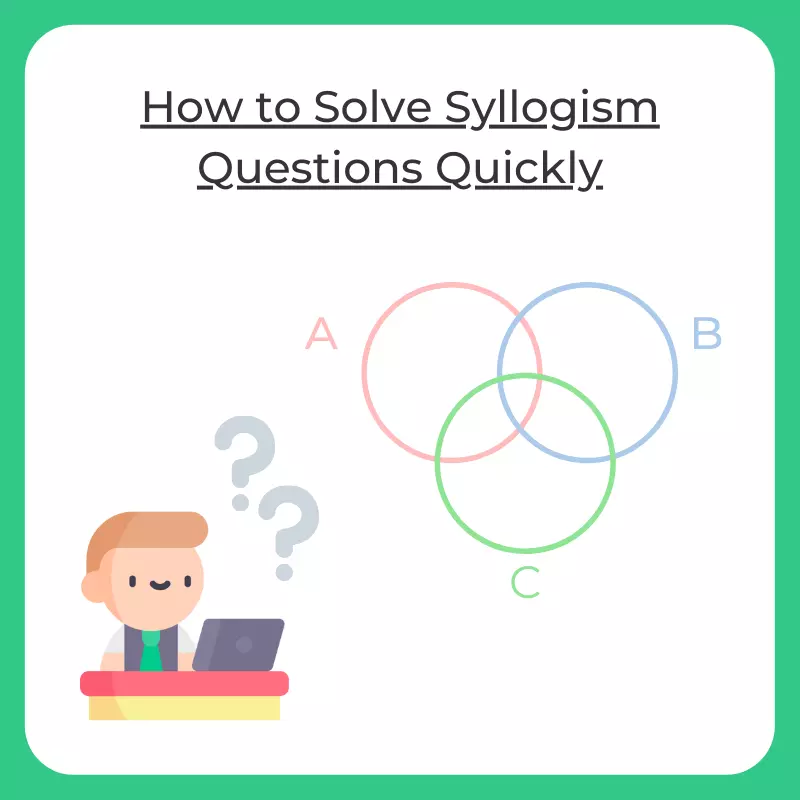## How to Solve Syllogism Questions Quickly

There are three different methods to solve Syllogims which are –

1. Verbal Method
2. Venn Diagram Method
3. Tick and Cross Method

Verbal Method –

It’s a very simple one, try to make logical deductions in your mind from Assumptions (Premises) and match which conclusion is true.

Example –

Premises

• All tigers are cats.
• All cats are animals.

From these, we can easily conclude that since all tigers are necessarily cats and all Cats are necessarily animals. So, all tigers are animals.

Conclusion- All Tigers are Animals.

Venn Diagram Method –

There are four categories to remember to solve using Venn’s Diagram.

#### Rule 1 Syllogism:

Statement – All A’s are B
Example – All Mangoes are Fruits

Conclusions that can be made out.

Conclusion 1 – Some A’s are B or Some Mangoes are Fruits
Conclusion 2 -Some B’s are A or Some Fruits are Mangoes.

Now, the first conclusion may confuse you as we said All A’s are B’s and then we are concluding some A’s are B’s.

In Syllogism its not wrong to say some A’s are B when you have a statement as All A’s are B’s, as –

Some A’s are actually B or Some Mangoes are actually fruit. This is not a wrong information#### Rule 2 Syllogism:

• Statement – No A is B
• Example – No Tomatoes are Fruits

This has a direct conclusion are No A is B

#### Rule 3 Syllogism:

These have these possible conclusions –

• Statement – Some A’s are B
• Example – Some men are Engineers

These have these possible conclusions –

1. Conclusion 1 – Some A are not B
2. Conclusion 2 – All A are B.
3. Conclusion 3 – All B are A
4. Conclusion 4 – All A are B and all B are A.Now, Conclusion 1 is clear as if some men are Engineers then some may not be engineers (Check Image for Conclusion below Conclusion 2 two is a case when we for a sample set, only Engineers maybe allowed to do engineering. Similar of Conclusion 3 For Conclusion 4 both men and Engineers are same sample set i.e Sample Set(Men) = Sample Set(Engineers)

### Related Banners

Get PrepInsta Prime & get Access to all 200+ courses offered by PrepInsta in One Subscription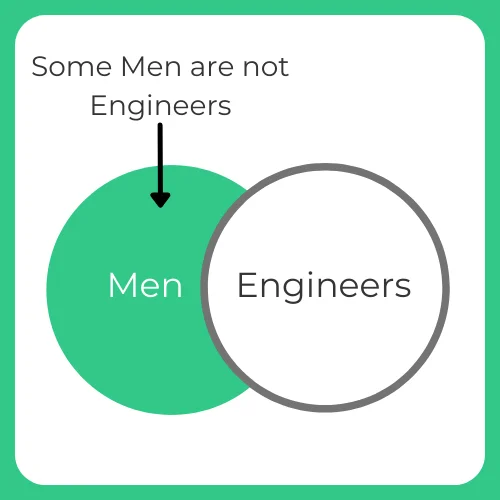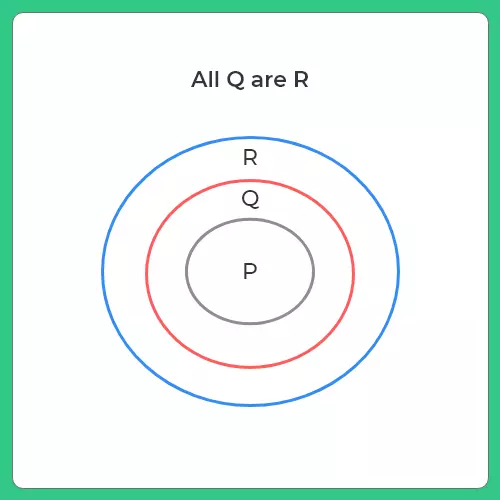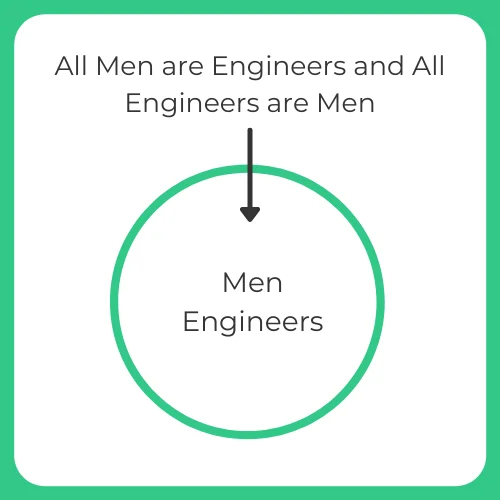#### Some handy How to Solve Syllogism Questions Quickly & Rules –

• All+All = All
• All+No = No
• All+Some = No Conclusion
• Some+All = Some
• Some+No =  Some Not
• Some+Some =  No Conclusion

If the conclusion provided in the question is in “Possibility” case then you must proceed as per the following rules:

1. If All A are B => Some B are Not A is a Possibility.
2. If Some B are Not A => All A are B is a Possibility.
3. If Some A are B => All A are B is a Possibility & All B are A is a Possibility.

## Summary of Venn Diagram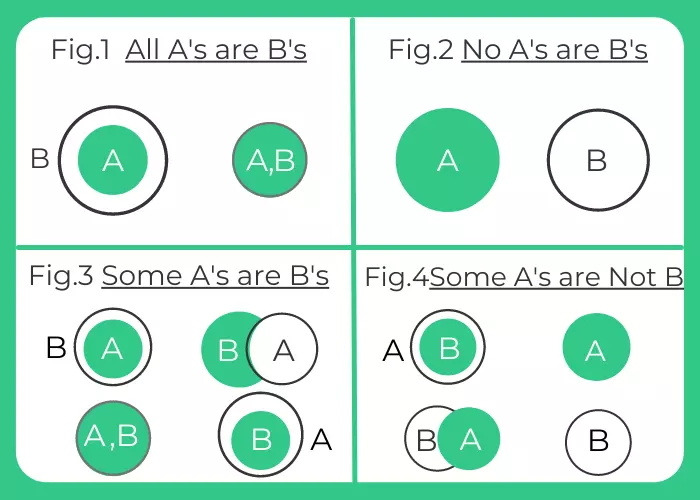Note: In a few questions drawing diagrams may be very cumbersome as you may have to draw 4 diagrams depending upon the possibilities arriving specially Rule 3 which has 4 possibilities.

## How to Solve Syllogism Questions Quickly

### Ticks and cross solves the problem of not having to create many diagrams.

Ticks – They denote defined set
Cross – They denote undefined set

Defined Set – When all the elements of that set have to be known in order to define a particular premise.

Undefined Set – If all the elements of a set need not be known in order to make a particular statement

Each Syllogism Premise must be one of the four –

 Universal Affirmative All As are Bs. Universal Negative No As are Bs. Particular Affirmative Some As are Bs. Particular Negative Some As are not Bs.

### Some Rules by Aristotle to solve Syllogism :

#### Checking if Conclusion is possible or not –

1. When Counting total premises and conclusion they should be 3
2. If we encounter a situation where in both the premises are negative then no conclusion can be achieved.
3. If we encounter a situation where in both the particular(some is used) are negative then no conclusion can be achieved.
4. The middle term (common term of both premises) must be distributed at least once.

#### Checking nature of conclusion –

1. If one premises is particular then conclusion will definitely be particular.
2. If one premises is negative then the conclusion will be negative.
3. A term that is not distributed in the premises cannot be distributed in the conclusion.
##### Learning with an Example –

Premises

• All parallelogram are four sided.
• Some parallelograms are square.

### Question 1.

Mentioned below are some statements followed by a conclusion in the options. Take the given statements to be true, even if they contradict to the commonly known facts, and determine the conclusions that logically follow the statements.

Statement:
1. All men are girls.
2. Some girls are students

Conclusion:
1. All girls are men
2. Some girls are not students.

Options:

A. The only Conclusion I follows

B. Only Conclusion II follows

C. Either conclusion I or Conclusion II follows

D. Neither conclusion I nor Conclusion II follows

#### Explanation:

Since both, the conclusions contain the term girls so neither of them can follow.

Hence option D is the correct one.

### Question 2.

Statement:
1.  All men are women.
2. All women are kids.

Conclusion:
1. All men are kids
2. Some men are not kids

Options:

A. The only Conclusion I follows

B. Only Conclusion II follows

C. Either conclusion I or Conclusion II follows

D. Neither conclusion I nor Conclusion II follows

#### Explanation:

By looking at the statements, it is evident that since all men are women and all women are kids, hence we can say that all men are kids. Thus it would be wrong to say that some men are not kids. Therefore the only conclusion I follow the statement.

Therefore one can say that option A is the correct one.

### Question 3.

Statement:
1. All Roses are flowers.
2. No Orchid is Lotus

Conclusion:
1. No Orchid is a flower
2. Some Orchids are flowers.

Options:

A. The only Conclusion I follows

B. Only Conclusion II follows

C. Either conclusion I or Conclusion II follows

D. Neither conclusion I nor Conclusion II follows

#### Explanation:

Here, the first statement is a universal positive statement, so the middle term ‘Roses is forming the predicate is distributed twice. So the conclusion cannot be universal. Therefore, either conclusion I or II must be followed.

## How to Solve Syllogism Questions Quickly (Venn Diagram method)

Another easier way to solve syllogism questions is with the help of the Venn diagram.

All we have to do is, read the given statements and accordingly draw the Diagram step by step, by the given statements, and eventually derive a logical solution with the help of the final diagram.

It can be explained in a better way with the help of a couple of examples below:

### Question 1.

Mentioned below are some statements followed by a conclusion in the options. Take the given statements to be true, even if they contradict to the commonly known facts, and determine the conclusions that logically follows the statements.

Statement:
1. No pen is coat.
2. No scales are pens.

Conclusion:
1. All scales are coat
2. Some scale is pen.

Options:

A. The only Conclusion I follows

B. Only Conclusion II follows

C. Either conclusion I or Conclusion II follows

D. Neither conclusion I nor Conclusion II follows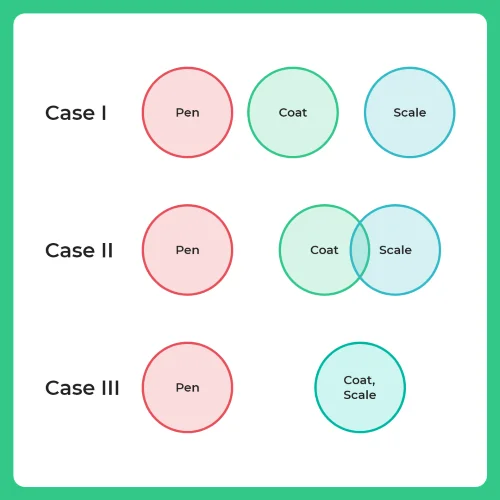#### Explanation

There are 3 possible cases

Since “ No pen is coat” The diagram of pen and coat do not have any overlapping. Hence, they are apart. According to the second statement, since no scales are pens, the diagrams of scales and pen do not overlap.

Case 1: If no scales are pen, one possibility is there can be no scales which is coat also.

Case 2: There can be scales which is also coat. Hence a part of scales and coat overlap with each other.

Case 3: All coats can be a scales, as there is no statement which says this combination is not possible.

Therefore option D is the correct one.

### Question 2.

Statement:
1. Some Jack is Jill
2. Some Max is Jack

Conclusion:
1. Some Jill is Max
2. No Jill is Max

Options:

A. The only Conclusion I follows

B. Only Conclusion II follows

C. Either conclusion I or Conclusion II follows

D. Neither conclusion I nor Conclusion II follows

#### Explanation: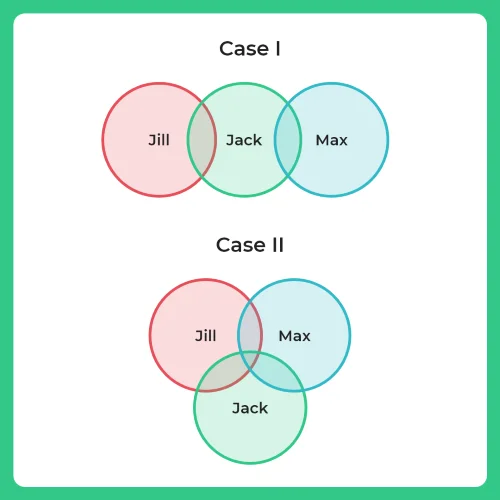By looking at the above diagram, it is evident that either conclusion I or conclusion II follow since the conclusion is forming a complementary pair.

Hence option C is the correct one.

### Question 3.

Statement:
1. All men are strong
2. Arpit is a man

Conclusion:
Arpit is strong
Arpit is not strong

Options:

A. The only Conclusion I follows

B. Only Conclusion II follows

C. Both conclusion I and Conclusion II follows

D. Neither conclusion I nor Conclusion II follows

#### Explanation: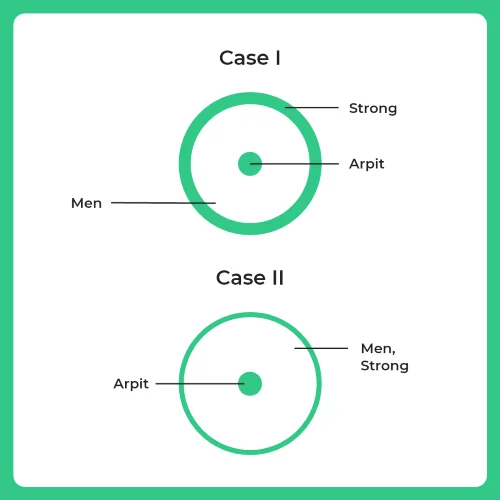In the first case, the statement “ All men are strong “ the Venn diagram of men is inside the Venn diagram of strong. In the second statement, since Arpit is a man, the Venn diagram representing Arpit should be inside man. In the second case, the only difference is, the first statement “ All men are strong “ the Venn diagrams of men and strong are overlapping with each other. Because that’s another possibility. Since Arpit is men, it is represented inside it. Observing both the cases, we can agree that the conclusion given “ Arpit is a strong “ is true from both the case.

Hence, Correct option A is the correct one.

## Get over 200+ course One Subscription

Courses like AI/ML, Cloud Computing, Ethical Hacking, C, C++, Java, Python, DSA (All Languages), Competitive Coding (All Languages), TCS, Infosys, Wipro, Amazon, DBMS, SQL and others

## Checkout list of all the video courses in PrepInsta Prime Subscription

### 3 comments on “How to Solve Syllogism Questions Quickly”

•Chandra

Statement:
1. Some Tigers are Rats
2. No Hens are Tigers

Conclusion:
No Tigers are Hens
Some Rats are Hens

Options:

A. The only Conclusion I follows

B. Only Conclusion II follows

C. Either conclusion I or Conclusion II follows

D. Neither conclusion I nor Conclusion II follows

Explanation:

Since the first conclusion contains the middle term ‘puppy’ as the subject and the second conclusion contains the middle terms ‘puppy’ as the predicate. Since the conclusion I am particular, and conclusion II is negative. So, no conclusion follows.
//nowhere is written about puppy and you are justifying the conclusion based upon puppy how?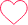5
•Chandra

nothing mentioned about rat and hen.. how u have drawn the venn diagram between them?10
•Chandra

Statement:
1. All men are girls.
2. Some girls are students

Conclusion:
1. All girls are men
2. Some girls are not students.

Options:

A. The only Conclusion I follows

B. Only Conclusion II follows

C. Either conclusion I or Conclusion II follows

D. Neither conclusion I nor Conclusion II follows

the answer should be both the conclusion 1 and 2 follows4